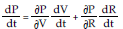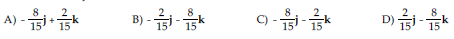/
/
/
181) Find ∂y/∂x at the point (1, 3, e8) for
Not my Question
Flag Content

# Question : 181) Find ∂y/∂x at the point (1, 3, e8) for : 1757199

181) Find y/x at the point (1, 3, e8) for ln(xz)y + 4y3 = 0.

A) -3/116 B) 116/3 C) 3/116 D) -116/3

182) A simple electrical circuit consists of a resistor connected between the terminals of a battery. The voltage V (in volts) is dropping as the battery wears out. At the same time, the resistance R (in ohms) is increasing as the resistor heats up. The power P (in watts) dissipated by the circuit is given by P = V2/R. Use the equationto find how much the power is changing at the instant when R = 5 ohms, V = 3 volts, dR/dt = 0.05 ohms/sec and dV/dt = -0.08 volts/sec.

A) 0.11 watts B) -0.11 watts C) -0.08 watts D) 0.08 watts

183) The radius r and height h of a cylinder are changing with time. At the instant in question, r = 5 cm, h = 3 cm, dr/dt = 0.03 cm/sec and dh/dt = -0.02 cm/sec. At what rate is the cylinder's volume changing at that instant?

A) 4.4 cm3/sec B) -0.16 cm3/sec C) 1.26 cm3/sec D) 1.1 cm3/sec

184) f(x, y) = 7x - 10y, (7, -5)

A) 42i + 50j B) 7i - 10j C) 6i - 5j D) 42i - 50j

185) f(x, y) = -9x2 + 8y, (-7, 3)

A) -441i + 24j B) 126i + 24j C) 126i + 8j D) -882i + 24j

186) f(x, y) = ln(10x + 9y), (-3, 2)

A) 1/4 i -1/6 j B) - 1/12 i - 1/12 j C) -1/6 i + 1/4 j D) -5/6 i -3/4 j

187) f(x, y) = tan-1 4x/y, (-2, 3)

A) 6/41 i + 4/41 j B) 12/73 i + 8/73 j C) 6/41 i - 4/41j D) 12/73 i - 8/73 j

188) f(x, y, z) = -2x - 4y + 7z, (9, -6, 3)

A) -18i - 24j + 21k B) 9i - 6j + 3k

C) -2i - 4j + 7k D) -18i + 24j + 21k

189) f(x, y, z) = 4xy3z2, (4, 64, 16)

A) 67,108,864i + 33,554,432j + 12,582,912k B) 268,435,456i + 50,331,648j + 134,217,728k

C) 67,108,864i + 50,331,648j + 8,388,608k D) 268,435,456i + 33,554,432j + 201,326,592k

190) f(x, y, z) = tan-1 3x/2y + 8z, (5, 0, 0)## Solution 5 (1 Ratings )

Solved
Mathematics 1 Month Ago 53 Views